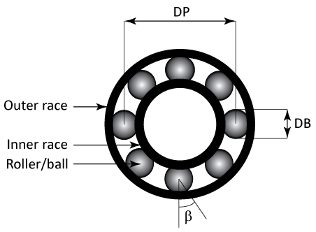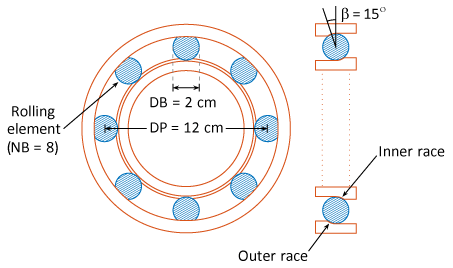# bearingFaultBands

Generate frequency bands around the characteristic fault frequencies of ball or roller bearings for spectral feature extraction

## Syntax

``FB = bearingFaultBands(FR,NB,DB,DP,beta)``
``FB = bearingFaultBands(___,Name,Value)``
``[FB,info] = bearingFaultBands(___)``
``bearingFaultBands(___)``

## Description

example

````FB = bearingFaultBands(FR,NB,DB,DP,beta)` generates characteristic fault frequency bands `FB` of a roller or ball bearing using its physical parameters. `FR` is the rotational speed of the shaft or inner race, `NB` is the number of balls or rollers, `DB` is the ball or roller diameter, `DP` is the pitch diameter, and `beta` is the contact angle in degrees. The values in `FB` have the same implicit units as `FR`.```

example

````FB = bearingFaultBands(___,Name,Value)` allows you to specify additional parameters using one or more name-value pair arguments.```

example

````[FB,info] = bearingFaultBands(___)` also returns the structure `info` containing information about the generated fault frequency bands `FB`.```

example

````bearingFaultBands(___)` with no output arguments plots a bar chart of the generated fault frequency bands `FB`.```

## Examples

collapse all

For this example, consider a bearing with a pitch diameter of 12 cm with eight rolling elements. Each rolling element has a diameter of 2 cm. The outer race remains stationary as the inner race is driven at 25 Hz. The contact angle of the rolling element is 15 degrees.With the above physical dimensions of the bearing, construct the frequency bands using `bearingFaultBands`.

```FR = 25; NB = 8; DB = 2; DP = 12; beta = 15; FB = bearingFaultBands(FR,NB,DB,DP,beta)```
```FB = 4×2 82.6512 85.1512 114.8488 117.3488 71.8062 74.3062 9.2377 11.7377 ```

`FB` is returned as a 4x2 array with default frequency band width of 10 percent of `FR` which is 2.5 Hz. The first column in `FB` contains the values of $F-\frac{W}{2}$, while the second column contains all the values of $F+\frac{W}{2}$ for each characteristic defect frequency.

For this example, consider a micro roller bearing with 11 rollers where each roller is 7.5 mm. The pitch diameter is 34 mm and the contact angle is 0 degrees. Assuming a shaft speed of 1800 rpm, construct frequency bands for the roller bearing. Specify '`Domain`' as '`frequency`' to obtain the frequency bands `FB` in the same units as `FR`.

```FR = 1800; NB = 11; DB = 7.5; DP = 34; beta = 0; [FB1,info1] = bearingFaultBands(FR,NB,DB,DP,beta,'Domain','frequency')```
```FB1 = 4×2 104 × 0.7626 0.7806 1.1994 1.2174 0.3791 0.3971 0.0611 0.0791 ```
```info1 = struct with fields: Centers: [7.7162e+03 1.2084e+04 3.8815e+03 701.4706] Labels: ["1Fo" "1Fi" "1Fb" "1Fc"] FaultGroups: [1 2 3 4] ```

Now, include the sidebands for the inner race and rolling element defect frequencies using the '`Sidebands`' name-value pair.

`[FB2,info2] = bearingFaultBands(FR,NB,DB,DP,beta,'Domain','order','Sidebands',0:1)`
```FB2 = 8×2 4.2368 4.3368 5.6632 5.7632 6.6632 6.7632 7.6632 7.7632 1.7167 1.8167 2.1064 2.2064 2.4961 2.5961 0.3397 0.4397 ```
```info2 = struct with fields: Centers: [4.2868 5.7132 6.7132 7.7132 1.7667 2.1564 2.5461 0.3897] Labels: [1x8 string] FaultGroups: [1 2 2 2 3 3 3 4] ```

You can use the generated fault bands `FB` to extract spectral metrics using the `faultBandMetrics` command.

For this example, consider a damaged bearing with a pitch diameter of 12 cm with eight rolling elements. Each rolling element has a diameter of 2 cm. The outer race remains stationary as the inner race is driven at 25 Hz. The contact angle of the rolling element is 15 degrees.With the above physical dimensions of the bearing, visualize the fault frequency bands using `bearingFaultBands`.

```FR = 25; NB = 8; DB = 2; DP = 12; beta = 15; bearingFaultBands(FR,NB,DB,DP,beta)```From the plot, observe the following bearing specific vibration frequencies:

• Cage defect frequency, `Fc` at 10.5 Hz.

• Ball defect frequency, `Fb` at 73 Hz.

• Outer race defect frequency, `Fo` at 83.9 Hz.

• Inner race defect frequency, `Fi` at 116.1 Hz.

For this example, consider a ball bearing with a pitch diameter of 12 cm with 10 rolling elements. Each rolling element has a diameter of 0.5 cm. The outer race remains stationary as the inner race is driven at 25 Hz. The contact angle of the ball is 0 degrees. The dataset `bearingData.mat` contains power spectral density (PSD) and its respective frequency data for the bearing vibration signal in a table.

First, construct the bearing frequency bands including the first 3 sidebands using the physical characteristics of the ball bearing.

```FR = 25; NB = 10; DB = 0.5; DP = 12; beta = 0; FB = bearingFaultBands(FR,NB,DB,DP,beta,'Sidebands',1:3)```
```FB = 14×2 118.5417 121.0417 53.9583 56.4583 78.9583 81.4583 103.9583 106.4583 153.9583 156.4583 178.9583 181.4583 203.9583 206.4583 262.2917 264.7917 274.2708 276.7708 286.2500 288.7500 ⋮ ```

`FB` is a 14x2 array which includes the primary frequencies and their sidebands.

Load the PSD data. `bearingData.mat` contains a table `X` where PSD is contained in the first column and the frequency grid is in the second column, as cell arrays respectively.

```load('bearingData.mat','X') X```
```X=1×2 table Var1 Var2 ________________ ________________ {12001x1 double} {12001x1 double} ```

Compute the spectral metrics using the PSD data in table `X` and the frequency bands in `FB`.

`spectralMetrics = faultBandMetrics(X,FB)`
```spectralMetrics=1×43 table PeakAmplitude1 PeakFrequency1 BandPower1 PeakAmplitude2 PeakFrequency2 BandPower2 PeakAmplitude3 PeakFrequency3 BandPower3 PeakAmplitude4 PeakFrequency4 BandPower4 PeakAmplitude5 PeakFrequency5 BandPower5 PeakAmplitude6 PeakFrequency6 BandPower6 PeakAmplitude7 PeakFrequency7 BandPower7 PeakAmplitude8 PeakFrequency8 BandPower8 PeakAmplitude9 PeakFrequency9 BandPower9 PeakAmplitude10 PeakFrequency10 BandPower10 PeakAmplitude11 PeakFrequency11 BandPower11 PeakAmplitude12 PeakFrequency12 BandPower12 PeakAmplitude13 PeakFrequency13 BandPower13 PeakAmplitude14 PeakFrequency14 BandPower14 TotalBandPower ______________ ______________ __________ ______________ ______________ __________ ______________ ______________ __________ ______________ ______________ __________ ______________ ______________ __________ ______________ ______________ __________ ______________ ______________ __________ ______________ ______________ __________ ______________ ______________ __________ _______________ _______________ ___________ _______________ _______________ ___________ _______________ _______________ ___________ _______________ _______________ ___________ _______________ _______________ ___________ ______________ 121 121 314.43 56.438 56.438 144.95 81.438 81.438 210.57 106.44 106.44 276.2 156.44 156.44 407.45 181.44 181.44 473.07 206.44 206.44 538.7 264.75 264.75 691.77 276.75 276.75 723.27 288.69 288.69 754.61 312.69 312.69 817.61 324.62 324.62 848.94 336.62 336.62 880.44 13.188 13.188 31.418 7113.4 ```

`spectralMetrics` is a 1x43 table with peak amplitude, peak frequency and band power calculated for each frequency range in `FB`. The last column in `spectralMetrics` is the total band power, computed across all 14 frequencies in `FB`.

## Input Arguments

collapse all

Rotational speed of the shaft or inner race, specified as a positive scalar. `FR` is the fundamental frequency around which `bearingFaultBands` generates the fault frequency bands. Specify `FR` either in Hertz or revolutions per minute.

Number of balls or rollers in the bearing, specified as a positive integer.

Diameter of the ball or roller, specified as a positive integer.

Pitch diameter of the bearing, specified as a positive scalar. `DP` is the diameter of the circle that the center of the ball or roller travels during the bearing rotation.

Contact angle in degrees between a plane perpendicular to the ball or roller axis and the line joining the two raceways, specified as a positive scalar.

### Name-Value Pair Arguments

Specify optional comma-separated pairs of `Name,Value` arguments. `Name` is the argument name and `Value` is the corresponding value. `Name` must appear inside quotes. You can specify several name and value pair arguments in any order as `Name1,Value1,...,NameN,ValueN`.

Example: `...,'Harmonics',[1,3,5]`

Harmonics of the fundamental frequency to be included, specified as the comma-separated pair consisting of '`Harmonics`' and a vector of positive integers. The default value is 1. Specify '`Harmonics`' when you want to construct the frequency bands with more harmonics of the fundamental frequency.

Sidebands around the fundamental frequency and its harmonics to be included, specified as the comma-separated pair consisting of '`Sidebands`' and a vector of nonnegative integers. The default value is 0. Specify '`Sidebands`' when you want to construct the frequency bands with sidebands around the fundamental frequency and its harmonics.

Width of the frequency bands centered at the nominal fault frequencies, specified as the comma-separated pair consisting of '`Width`' and a positive scalar. The default value is 10 percent of the fundamental frequency. Avoid specifying '`Width`' with a large value so that the fault bands do not overlap.

Units of the fault band frequencies, specified as the comma-separated pair consisting of '`Domain`' and either `'frequency'` or `'order'`. Select:

• `'frequency'` if you want `FB` to be returned in the same units as `FR`.

• `'order'` if you want `FB` to be returned as number of rotations relative to the inner race rotation, `FR`.

## Output Arguments

collapse all

Fault frequency bands, returned as an `Nx2` array, where `N` is the number of fault frequencies. `FB` is returned in the same units as `FR`, in either hertz or orders depending on the value of '`Domain`'. Use the generated fault frequency bands to extract spectral metrics using `faultBandMetrics`. The generated fault bands, , are centered at:

• Outer race defect frequency, `Fo` and its harmonics

• Inner race defect frequency, `Fi`, its harmonics and sidebands at `FR`

• Rolling element (ball) defect frequency, `Fb`its harmonics and sidebands at `Fc`

• Cage (train) defect frequency, `Fc` and its harmonics

The value `W` is the width of the frequency bands, which you can specify using the '`Width`' name-value pair. For more information on bearing frequencies, see Algorithms.

Information about the fault frequency bands in `FB`, returned as a structure with the following fields:

• `Centers` — Center fault frequencies

• `Labels` — Labels describing each frequency

• `FaultGroups` — Fault group numbers identifying related fault frequencies

## Algorithms

`bearingFaultBands` computes the different characteristic bearing frequencies as follows:

• Outer race defect frequency, ${F}_{o}=\frac{NB}{2}FR\left(1-\frac{DB}{DP}\text{cos}\left(\beta \right)\right)$

• Inner race defect frequency, ${F}_{i}=\frac{NB}{2}FR\left(1+\frac{DB}{DP}\text{cos}\left(\beta \right)\right)$

• Rolling element (ball) defect frequency, ${F}_{b}=\frac{DP}{2DB}FR\left(1-{\left[\frac{DB}{DP}\text{cos}\left(\beta \right)\right]}^{2}\right)$

• Cage (train) defect frequency,${F}_{c}=\frac{FR}{2}\left(1-\frac{DB}{DP}\text{cos}\left(\beta \right)\right)$

 Chandravanshi, M & Poddar, Surojit. "Ball Bearing Fault Detection Using Vibration Parameters." International Journal of Engineering Research & Technology. 2. 2013.

 Singh, Sukhjeet & Kumar, Amit & Kumar, Navin. "Motor Current Signature Analysis for Bearing Fault Detection in Mechanical Systems." Procedia Materials Science. 6. 171–177. 10.1016/j.mspro.2014.07.021. 2014.

 Roque, Antonio & Silva, Tiago & Calado, João & Dias, J. "An approach to fault diagnosis of rolling bearings." WSEAS Transactions on Systems and Control. 4. 2009.